Click to Chat

1800-1023-196

+91-120-4616500

CART 0

• 0

MY CART (5)

Use Coupon: CART20 and get 20% off on all online Study Material

ITEM
DETAILS
MRP
DISCOUNT
FINAL PRICE
Total Price: Rs.

There are no items in this cart.
Continue Shopping• Complete JEE Main/Advanced Course and Test Series
• OFFERED PRICE: Rs. 15,900
• View Details

```Chapter 5: Operations on Rational Numbers Exercise – 5.1

Question: 1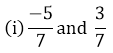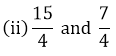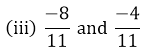Solution:We have,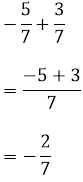We have,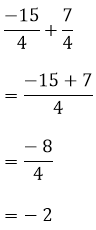We have,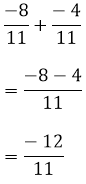We have,Question: 2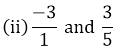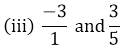Solution:If p/q and r/s are two rational numbers such that q and s do not have a common factor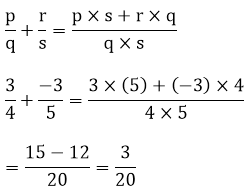If p/q and r/s are two rational numbers such that q and s do not have a common factor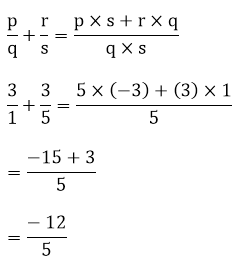LCM of 27 and 18 is 54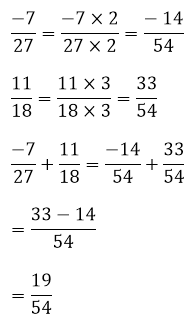LCM of 4 and 8 is 4Question: 3

Simplify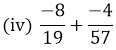Solution: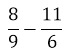LCM of 5 and 10 is 10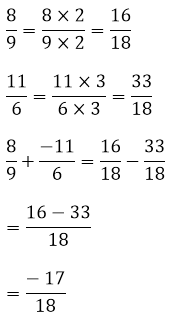LCM of 16 and 24 is 48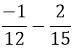LCM of 12 and 15 is 60LCM of 19 and 57 is 57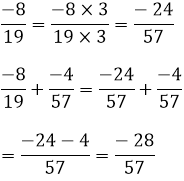Question: 4

Add and express the sum as a mixed fraction: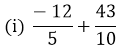Solution:LCM of 5 and 10 is 10LCM of 7 and 4 is 28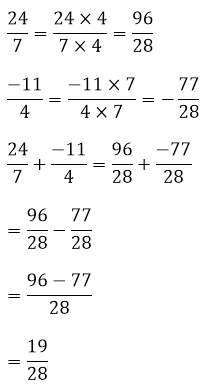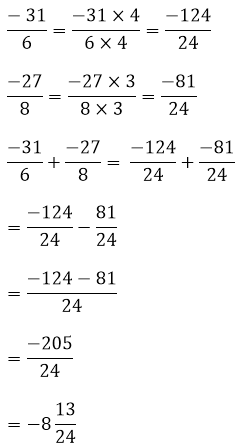```### Course Features

• 728 Video Lectures
• Revision Notes
• Previous Year Papers
• Mind Map
• Study Planner
• NCERT Solutions
• Discussion Forum
• Test paper with Video Solution